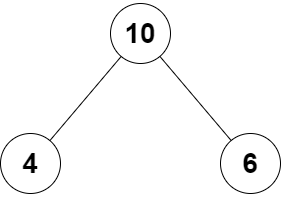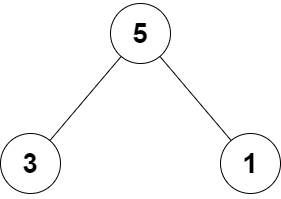# 2236. Root Equals Sum of Children

Easy
You are given the `root` of a binary tree that consists of exactly `3` nodes: the root, its left child, and its right child.
Return `true` if the value of the root is equal to the sum of the values of its two children, or `false` otherwise.
Example 1:Input: root = [10,4,6]
Output:
true
Explanation:
The values of the root, its left child, and its right child are 10, 4, and 6, respectively.
10 is equal to 4 + 6, so we return true.
Example 2:Input: root = [5,3,1]
Output:
false
Explanation:
The values of the root, its left child, and its right child are 5, 3, and 1, respectively.
5 is not equal to 3 + 1, so we return false.
Constraints:
• The tree consists only of the root, its left child, and its right child.
• `-100 <= Node.val <= 100`

### 解題

Runtime: 0 ms, faster than 100.00%
Memory Usage: 2 MB, less than 95.31%
/**
* Definition for a binary tree node.
* type TreeNode struct {
* Val int
* Left *TreeNode
* Right *TreeNode
* }
*/
func checkTree(root *TreeNode) bool {
return root.Val == root.Left.Val + root.Right.Val
}